﻿ Analysis and Design of a Three Inputs Fuzzy System for Maintaining the Cane Level during Sugar Manufacturing

### Analysis and Design of a Three Inputs Fuzzy System for Maintaining the Cane Level during Sugar Manuf...

Yogesh Misra, H R KamathOPEN ACCESSPEER-REVIEWED

## Analysis and Design of a Three Inputs Fuzzy System for Maintaining the Cane Level during Sugar Manufacturing

Yogesh Misra1,, H R Kamath2

1Mewar University, Chittorgarh, Rajasthan, India

### Abstract

The amount of cane fiber carried by cane carrier in a sugar mill varies due to non-uniformity of cane supply. The continuous variation of cane in Donnelly chute during the cane juice extraction inversely affects the cane juice extraction efficiency of mill. This paper deals with the development of algorithm for a three input fuzzy controller with an aim to maintain the cane level in Donnelly chute during cane juice extraction. The developed controller generates the required rake carrier motor speed in rpm depending upon the value of cane level in Donnelly chute, quantity of cane on rake carrier and the roll speed. The three inputs fuzzy controller is developed and simulated for six cases by using fuzzy logic toolbox of ‘MATLAB® version 7.11.0.584 (R2020b).

### At a glance: Figures

• Misra, Yogesh, and H R Kamath. "Analysis and Design of a Three Inputs Fuzzy System for Maintaining the Cane Level during Sugar Manufacturing." Journal of Automation and Control 2.3 (2014): 62-78.
• Misra, Y. , & Kamath, H. R. (2014). Analysis and Design of a Three Inputs Fuzzy System for Maintaining the Cane Level during Sugar Manufacturing. Journal of Automation and Control, 2(3), 62-78.
• Misra, Yogesh, and H R Kamath. "Analysis and Design of a Three Inputs Fuzzy System for Maintaining the Cane Level during Sugar Manufacturing." Journal of Automation and Control 2, no. 3 (2014): 62-78.

 Import into BibTeX Import into EndNote Import into RefMan Import into RefWorks

### 1. Introduction

Sugar is one of the oldest commodities originated from India in 4th century AD. Indians first discovered how to manufacture sugar. Now a days the sugar industry processes sugar cane and sugar beet to manufacture edible sugar. More than sixty percent of the world’s sugar production is from sugar cane; the balance is from sugar beet . Sugarcane plays a very important role in the economy of Indian farmers as it is the only input used by sugar mills to produce sugar in India. There is similar process for sugar making adopted in the entire sugar mill in India.

The sugar making process flow is shown in Figure 1. During sugar manufacturing the cane billets are converted into cane fiber and this cane fiber is crushed for the extraction of cane juice. The residual of fiber after juice extraction is used as fuel in boiler house for the generation of steam. The cane juice extracted contains impurities and the clarification of the cane juice is the next step. The juice clarification process converts the impurities into a thick paste of mud which settle down in the clarifier vessel while clarified juice overflows the clarifier vessel. The separated mud is called as filter cake and used as fertilizers. The thin clarified juice is concentrate to heavy syrup by boiling it from a series of evaporators at high vacuum. The heavy syrup contains 65% of sugar. The heavy syrup, which is also called as mother liquor, is seeded with small sugar crystal and further concentrated by boiling in vacuum pan. This process of crystal growth by adding seed crystal in syrup is called crystallization. The mixture of mother liquor and grown crystals in pan is called as massecuite. Crystals of required size are grown typically in four hours. After the growth of sugar crystals the massecuite is discharged in receiving tank. The mother liquor separated from sugar crystals during centrifugal process is called as molasses. The raw sugar from the centrifugal is dried by tumbling through a stream of air in a rotating drum. The dried sugar is then stored and ready to use [2, 3].

Cane juice extraction is a very important process during sugar making. The supply of cane for processing is very uneven and this uneven supply of cane during juice extraction adversely affects the sugar mill efficiency and causes mill breakdown, stoppage and jamming .

In this paper we have developed an intelligent three input fuzzy controller which maintains the cane level at desired height in Donnelly chute during cane crushing by nullifying the variation in cane supply and roll speed. The fuzzy controller for maintaining the cane level in Donnelly chute generates the signal for controlling the speed of motor driving the rake carrier depending upon the cane amount on rake, level of cane in Donnelly chute and roll speed.

In Section II we have discussed the cane juice extraction process and some important terminology used in sugar mills. In Section-III we discussed the three inputs fuzzy controller design philosophy and algorithm. The three inputs fuzzy controller is simulated for six different cases by using fuzzy toolbox of ‘MATLAB® version 7.11.0.584 (R2020b). In Section-IV the simulation results of the fuzzy controller is discussed and finally Section-V contains the concluding remark.

### 2. Cane Juice Extraction and Important Mill Terminology

A schematic of cane juice extraction process is shown in Figure 2. Cane juice extraction is very important process of a sugar mill which affects the sugar mill’s efficiency. The cane billets received by sugar mill from cane growers are weighted and dumped in cane yard. From the cane yard the cane billets are dumped in the cane carrier with the help of a crane. The cane carrier moves continuously and is responsible for bringing the cane to the factory for sugar production. The cane is first passed through two sets of rotating knives. The first one is called cane knives and it cut the cane into pieces. The second set is called as shredder knives and it prepare the fiber of cane billets. The small fibers are around 1-2 cm. The cane fibers are feed to Donnelly chute via a rake carrier and cane juice is extracted by crushing fiber in two or three rolls of the mill. This process is repeated through sets of five/six mills until last mill is reached. Before the crushing of cane fiber in last mill it is immersed in a diluted mixture of final mill cane crushing juice and hot water. The residual cane fiber after the last mill is called bagasse is conveyed to bagasse bin and finally to the boiler station where it is used as fuel [2, 3, 4].

In a sugar mill for optimum juice extraction and better sugar production efficiency the cane level in Donnelly chute is to be maintained constant. The height of cane level above the mill depends upon various parameters like roll diameter, work opening, surface speed of roll, feed depth, contact angle between the roll, escribed volume and chute inclination with respect to horizontal.

Cane fiber is carried by rake carrier and finally dumped in Donnelly chute from where it is crushed by the rollers. The amount of cane fiber carried by rake carrier varies due to non-uniformity of cane supply. If the level of cane fiber falls below the desired level then the speed of rake carrier motor is to be increased and if the level of cane fiber rises above the desired level the speed of rake carrier motor is to be decreased for maintaining the desired level. The variation of desired level of cane in chute is also affected by the variation of roll surface speed. The steam turbines are used to rotate the rolls in sugar mill. The final product left out after the extraction of juice from the milling train is called baggasse. This baggase is used as fuel to produce steam and this steam is used to run turbines. In a sugar mill the supply of steam to run turbine is not uniform therefore the rotational speed of rolls vary.

Two rolls and the chute arrangement used for cane crushing are shown in Figure 3. It has been investigated that the physical structure of mill effect the feed depth at which maximum crushing rate can be achieved . The diameter of roll when measured from the tip of groves is Do and Dg is the length of groves and D is the average diameter of roll. The mean diameter of roll is given as:(1)

Where Do is the outside diameter of roll and Dg is Groove Depth. The opening measured between the two rolls outside diameter is called as nib opening or set opening. The opening measured between the mean diameters of two rolls is called work opening and is given as:(2)

Where Ws is nib opening. The surface speed of roll is given as:(3)

Where S is surface speed of roll in cm/s and N is roll shaft speed in rpm. The thickness of cane blanket at the feed opening of the mill effects the juice extraction from the mill. The optimum feed depth is investigated and found as follows:(5)

Where Bc is the optimum feed depth in cm . The contact angle is the angle between the line joining the center of the two rolls and the line joining the center of roll to the point where chute touches the roll . The contact angle is given as: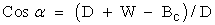(6)

The escribed volume is the volume of prepared cane passing through the work opening of the mill  and is given as: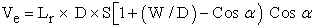(7)

Where Ve is escribed volume in (m3/s) and Lr is roll length in cm. The average speed of cane blanket at the point where chute touches the rolls is given as:(8)

Where Sf is the average speed of cane blanket in cm/s. At the entry of chute the volume of cane passing the entry plane is given as :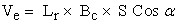(9)

The fibre rate is the amount of fibre crushed by mill in one second and it is given as: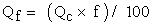(10)

Where Qf is the fibre rate in Kg/s, Qc is cane crushing rate in Kg/s and f is the percentage of fibre present in cane [6, 7].

The prepared cane is carried by cane carrier and dumped in chute. The chute is inclined to horizontal and the angle of inclination and the dimension of chute vary from one mill to other. The length of chute (Lc) is 180cm, width (Wc) is 43.5cm and depth (Dc) is 183cm. The Roll length (Lr) is 183cm, roll diameter (D) is 75.5cm and work opening (W) is 11.45cm. The optimum angle (α) calculated from (6) is 61°. The optimum feed depth (Bc) calculated from (5) is 43.5cm.

It is required to select parameters for a mill which can crush 2000 tons cane per day (tcd). Allowing about 10% excess crushing, the maximum mill capacity should be 2200 tcd. For achieving 2200 tcd crushing of cane the mill must be able to crush 26.6Kg/s cane . The amount of cane crushed by mill in one second is termed as flow rate and denoted as Qc in this paper. We can relate flow rate with cane density and escribed volume as follows: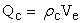(11)

Where Ve is escribed volume and ρc is density of cane (350Kg/m3). The escribed volume calculated from (11) is 0.076m3 /s. The surface speed of roll is calculated from (9) is 16.6cm/s. The average speed of cane blanket when it touches the roll surface is calculated from (8) is 9.5cm/s. If the cane crushing rate is 26Kg/s and the fibre percentage in cane is 15% then the fibre rate calculated from (10) is 4Kg/s. The Pressure required to feed mill is given as follows :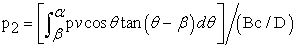(12)

Where p2 is the pressure required at chute exit, pv is the pressure applied to the cane, θ is the angle with which chute is inclined to horizontal, β is the angle with which mill will be feed without the application of external force . β is given as:(13)

Where µ is the coefficient of friction and its value selected in this application is 0.3. Solving (12) after putting α = 61°, θ=61° and β = 20° gives as:(14)

The pv is given as:(15)

Where Cf is filling ratio and is given as:(16)

Where γ is called compaction  and its value in this application is 52Kg/m3. The value of Cf calculated from (16) is 0.041. The value of the pressure applied to the cane (pv) is calculated as 23.6lb/ft2 (0.01152Kg-force/cm2) and the pressure required at chute exit (p2) is calculated as 0.02352Kg-force/cm2 (= 2.3KPa). In an open chute, the pressure due to fiber is given as:(17)

Where L is the height of cane in chute and the value of θ in this application is 61°. The height of cane in open chute is calculated from (17) as 92cm. In order to minimize the failure rate of fiber the cane must be maintained at 90cm in chute. The cane fiber is assumed to fail in a similar was to soils. The ‘failure ratio’  in fiber is the ratio of maximum shear stress to shear strength of fiber. A volume of prepared cane contains fiber, air and juice. When fiber is compressed in a pair of roll then air is expressed until fiber contains only fiber and juice. Any further compression of fiber expresses juice. It has been investigated that failure rate of fiber decreases with the increment of pressure applied on fiber at mill opening but beyond certain value the failure rate starts increasing with the increment of pressure. The failure rate is minimum (0.04) when the feed pressure is 2kPa .

### 3. Three Inputs Fuzzy Controller Design Philosophy and Algorithm

The proposed three input fuzzy controller based system to control the cane level is shown in Figure 4. The three variables in juice extraction process are weight of prepared cane on rake carrier, level of cane in Donnelly chute and the rotational speed of rolls. The prepared cane is dumped in Donnelly chute of height 180cm. The Rake Carrier which carries the prepared cane up to Donnelly chute is of length 800cm, width 150cm and its weight is 500Kg. The rake carrier is run by a motor whose speed can be varied from 17rpm to 116rpm. The amount of prepared cane on rake carrier varies from 500Kg to 1000Kg. This variation of prepared cane can be measured with a load cell. Due to uneven supply of cane billets the level of prepared cane varies in Donnelly chute. This variation of cane level is measured with the help of a light sensor. The two rolls rotate in anti-clockwise direction with the surface speed in the range from 12cm/s to 16.6cm/s. The steam turbines are used to rotate the rolls in sugar mill. In a sugar mill the supply of steam to run turbine is not uniform therefore the rotational speed of rolls vary. A tachometer can be used to calculate the speed of rolls. The details of interfacing circuits with the fuzzy controller are not covered in this paper. Rather we are concentrating on the development of three inputs fuzzy control algorithm with an aim of changing the speed of rake carrier depending upon the values of the three variables so that the cane level in Donnelly chute will remain constant. It has been calculated that for maintaining the 2200tcd crushing the flow rate should be 26.6Kg/cm. It has also been calculated that for achieving 26.6Kg/s flow rate the roll surface speed should be 16.6cm/s. If we assume that the roll speed will remain same then depending on the height of cane in chute and amount of cane available in rake carrier the speed of rake carrier is controlled to vary the feed rate which ultimately maintains the cane level at 90cm. If due to the variation in steam pressure the roll speed vary then the flow rate will also change and controller must be able to nullify these variations so that the cane level should remain constant at 90cm.

In order to design a Fuzzy Inference System (FIS) to control the level of cane fibers in Donnelly chute, we must describe the operation of system linguistically.

The four main components of a FIS [13, 14] are as follows:

i. Fuzzifier – It performs the function of fuzzification. During fuzzification the crisp inputs (input values from real world) is converted into a set of membership values in the interval [0,1] in the corresponding fuzzy sets.

ii. Rule Base – In the rule base the fuzzy inputs are compared based on the membership functions of each input and the rule base developed.

iii. Inference Engine – It generates the output of the fuzzy controller into linguistic variable.

iv. Defuzzifier – It performs the function of defuzzification. During defuzzification the fuzzy outputs of the inference engine is again converted into crisp form so that it can be used in real world [12, 13].

The input (crisp or numerical) data are fed into fuzzy logic rule based system where physical quantities are represented into linguistic variables with appropriate membership functions. These linguistic variables are then used in the (IF-Part) of a set of fuzzy. “IF THEN” rules within an inference engine to result in a new set of fuzzy linguistic variables or consequent (THEN-Part). Fuzzifier maps crisp numbers into fuzzy sets. Fuzzifier is used to activate the particular rules having the linguistic variable associated with specific fuzzy set. Inference Engine work just as we humans think before coming to decisions.

A text block of FIS is shown in Figure 5. Three input parameters and one output parameters selected for the modeling of fuzzy controller are ‘HEIGHT’, ‘WEIGHT’, ‘ROLLSPEED’ and ‘SPEED’ respectively. All the membership functions in input and output parameters in this Algorithm are triangular.

Input parameter ‘HEIGHT’ represents the level of prepared cane in Donnelly Chute in centimeter, ‘WEIGHT’ represents the weight of cane in cane carrier in Kilogram and ‘ROLLSPEED’ represents the speed of roll of the mill in cm/s. The output parameter ‘SPEED’ represents the speed of the rake carrier motor in rpm.

A. Fuzzification of ‘HEIGHT’

The universe of discourse of input parameter ‘HEIGHT’ is in the range 0 to 180cm and it is fuzzified into seven triangular linguistic variables as shown in Figure 6 and represented in Table 1. It has been calculated that the level of cane in the chute should be maintained at 90cm for proper feeding of prepared cane in the mill. Therefore during the selection of membership function it is decided that the 90cm height of cane in chute must possess membership value 1 for linguistic variable JR (Just Right).

#### Table 1. Linguistic Variables of Input Parameter ‘HEIGHT

B. Fuzzification of ‘WEIGHT’

The universe of discourse of input parameter ‘WEIGHT’ is in the range 500Kg to 1000Kg and it is fuzzified into eleven triangular linguistic variables as shown in Figure 7 and represented in Table 2.

C. Fuzzification of ‘ROLLSPEED’

The universe of discourse of input parameter ‘ROLLSPEED’ is in the range 12cm/s to 16.6cm/s and it is fuzzified into three triangular linguistic variables as shown in Figure 8 and represented in Table 3. The triangular membership functions of each linguistic variable of all input parameters are selected such that each one overlaps 50% with its neighbor.

#### Table 3. Linguistic Variables of Input Parameter ‘ROLLSPEED

D. Fuzzy Rule Base Development Algorithm

The algorithm used to develop the rule base of proposed fuzzy controller is shown in Figure 9. Two hundred thirty one rules are defined for the proposed fuzzy controller which generates the value at which the rake carrier motor should run for maintaining the cane level at 90cm height. The fuzzy rules are developed for three conditions of roll speed i.e. when the roll speed is 12cm/s (RL), 14.3cm/s (RM) and 16.6cm/s (RR).

When the cane level in chute is at 0cm, 30cm and 60cm then the feed rate of cane is increased by 42%, 31% and 20% of flow rate respectively. If the cane level in chute is at 90cm then the feed rate of cane should be same as flow rate of cane. If the cane level in chute is at 120cm, 150cm and 180cm then the feed rate is decreased by 20%, 31% and 42% of flow rate respectively. The rule base development matrix when roll speed is 12cm/s (RL), 14.3cm/s and 16.6cm/s are shown in Table 4, Table 5 and Table 6 respectively.

#### Table 6. Three Inputs Fuzzy Controller Design Algorithm When the Roll Speed is 16.6cm/s

E. Flow Rate Calculations for 12.0cm/s Roll Speed

When the surface speed of roll is 12.0cm/s then average speed of cane blanket when it touches the roll surface (Sf) is 6.9cm/s from (8). The escribed volume (Ve) at the entry of chute is 0.055m3/s from (9). The mass flow rate of cane through the rolls (Qc) is 19.3Kg/s from (11) and the fibre rate of cane through the rolls (Qf) from (10) when cane has 15% fibre percentage is 2.9Kg/s.

F. Flow Rate Calculations for 14.3cm/s Roll Speed

When the surface speed of roll is 14.3cm/s then average speed of cane blanket when it touches the roll surface (Sf) is 8.2cm/s from (8). The escribed volume (Ve) at the entry of chute is 0.065m3/s from (9). The mass flow rate of cane through the rolls (Qc) is 22.8Kg/s from (11) and the fibre rate of cane through the rolls from (10) is 3.5Kg/s.

G. Flow Rate Calculations for 16.6cm/s Roll Speed

When the surface speed of roll is 16.6cm/s then average speed of cane blanket when it touches the roll surface (Sf) is 9.5cm/s from (8). The escribed volume (Ve) at the entry of chute is 0.076m3/s from (9). The mass flow rate of cane through the rolls (Qc) is 26.6Kg/s from (11) and the fibre rate of cane through the rolls from (10) is 4.0Kg/s.

H. Fuzzification of ‘SPEED’

The universe of discourse of output parameter ‘SPEED’ is in the range 17.2rpm to 115.5rpm and it is fuzzified into nine triangular linguistic variables as shown in Figure 10 and represented in Table 7. It has been calculated that for maintaining 2,200tcd the roll speed should be 16.6cm/s. It is shown in S. No. 39 of Table 6 that for 750Kg of cane in rake the rake carrier motor should run at 54.2rpm to maintain cane level at 90cm. Therefore during the selection of membership function it is decided that the 54.2rpm must possess membership value 1 for linguistic variable JR (Just Right).

#### Table 7. Linguistic Variables of Output Parameter ‘SPEED”

The surface viewer of FIS representing the relationship between input parameters ‘HEIGHT’ and ‘WEIGHT’ and output parameter ‘SPEED’ is shown in Figure 11. The surface viewer of FIS representing the relationship between input parameters ‘ROLLSPEED’ and ‘HEIGHT’ and output parameter ‘SPEED’ is shown in Figure 12. The surface viewer of FIS representing the relationship between input parameters ‘WEIGHT’ and ‘ROLLSPEED’ and output parameter ‘SPEED’ is shown in Figure 13.

I. Explanation of S. No. 1 of Table 4

The cane level of 0cm in Donnelly chute, prepared cane of 500Kg in rake carrier and roll speed of 12cm/s are represented by EL, SL and RL linguistic variables of input parameters ‘HEIGHT’, ‘WEIGHT’ and ‘ROLLSPEED’ respectively. It has been calculated that if the roll speed is 12cm/s then the flow rate of cane through the rolls will be 19.3Kg/s. According to the algorithm under these conditions the feed rate should be increased by 42% of flow rate and it comes to be 27.4Kg/s. The weight of cane in 1cm of rake carrier when it has 500Kg cane is 0.625Kg. The carrier speed is calculated from (9) which comes to be 43.8cm/s and motor speed in rpm to run carrier at 43.8cm/s is calculated from (11) which comes to be 83.7rpm.

J. Explanation of S. No. 39 of Table 5

The cane level of 90cm in Donnelly chute, prepared cane of 750Kg in rake carrier and roll speed of 14.3cm/s are represented by JR, JR and RM linguistic variables of input parameters ‘HEIGHT’, ‘WEIGHT’ and ‘ROLLSPEED’ respectively. It has been calculated that if the roll speed is 14.3cm/s then the flow rate of cane through the rolls will be 22.8Kg/s. According to the algorithm under these conditions the feed rate should be equal to the flow rate. The weight of cane in 1cm of rake carrier when it has 750Kg cane is 0.938Kg. The carrier speed is calculated from (9) which comes to be 24.3cm/s and motor speed in rpm to run carrier at 24.3cm/s is calculated from (11) which comes to be 46.4rpm.

K. Explanation of S. No. 57 of Table 6

The cane level of 150cm in Donnelly chute, prepared cane of 550Kg in rake carrier and roll speed of 16.6cm/s are represented by VH, UL and RR linguistic variables of input parameters ‘HEIGHT’, ‘WEIGHT’ and ‘ROLLSPEED’ respectively. It has been calculated that if the roll speed is 16.6cm/s then the flow rate of cane through the rolls will be 26.6Kg/s. According to the algorithm under these conditions the feed rate should be decreased by 31% of flow rate and it comes to be 18.4Kg/s. The weight of cane in 1cm of rake carrier when it has 550Kg cane is 0.688Kg. The carrier speed is calculated from (9) which comes to be 26.7cm/s and motor speed in rpm to run carrier at 26.7cm/s is calculated from (11) which comes to be 51.0rpm.

L. Examples of Rule Base Development

Example-1: Rule 1 Antecedent is “If (HEIGHT is EL) and (WEIGHT is SL) and (ROLLSPEED is RL)”

For the antecedent of Rule 1 the motor speed should be 83.7rpm (S. No. 1 of Table 4). The motor speed 83.7rpm lies in VH and EH linguistic variable of ‘SPEED’ but it’s membership value is higher in VH as compared to EH (Figure 10.) therefore the consequent of Rule 1 is “then (SPEED is VH)”. So, the antecedent and consequent of Rule 1 is given as:

Rule 1: If (HEIGHT is EL) and (WEIGHT is SL) and (ROLLSPEED is RL) then (SPEED is VH)

Example II: Rule 91 Antecedent is “If (HEIGHT is VL) and (WEIGHT is EL) and (ROLLSPEED is RM)”

For the antecedent of Rule 91 the motor speed should be 76.2rpm (S. No. 14 of Table 5). The motor speed 76.2rpm lies in H and VH linguistic variable of ‘SPEED’ but it’s membership value is higher in VH as compared to H (Figure 10.) therefore the consequent of Rule 91 is “then (SPEED is VH)”. So, the antecedent and consequent of Rule 91 is given as:

Rule 91: If (HEIGHT is VL) and (WEIGHT is EL) and (ROLLSPEED is RM) then (SPEED is VH)

Example III: Rule 201 Antecedent is “If (HEIGHT is H) and (WEIGHT is EL) and (ROLLSPEED is RR)”

For the antecedent of Rule 201 the motor speed should be 54.2rpm (S. No. 47 of Table 6). The motor speed 54.2rpm lies in L and JR linguistic variable of ‘SPEED’ but it’s membership value is higher in JR as compared to H (Figure 10) therefore the consequent of Rule 201 is “then (SPEED is JR)”. So, the antecedent and consequent of Rule 91 is given as:

Rule 201: If (HEIGHT is H) and (WEIGHT is EL) and (ROLLSPEED is RR) then (SPEED is JR)

### 4. Result and Discussion

Three inputs fuzzy controller developed is simulated for six different cases by using fuzzy toolbox of ‘MATLAB® version 7.11.0.584 (R2020b). The controller generates the required rake carrier motor speed in rpm depending upon the value of cane level in chute, quantity of cane on rake carrier and the roll speed. The sampling period is 10 seconds and total simulation duration is 210 seconds.

In Case-I and Case-II the cane level and cane weight on carrier at the start of simulation is 90cm and 750Kg respectively. The roll speed at the start of simulation is 15cm/s for Case-I and 15.4cm/s for Case-II. The roll speed variation is less in Case-I and in Case-II it varies at the time of each sampling. The Matlab fuzzy tool box simulation result for specified condition for Case-I and Case-II is shown in Figure 14 and Figure 15 respectively. The simulation result is given in Table 11 and Table 12, the graphical representation of simulation result is given in Figure 20 and Figure 21 for Case-I and Case-II respectively.

#### Table 12. Case-II Cane Level is at 90cm and Roll Speed Vary during Each Sample

In Case-III and Case-IV the cane level at the start of simulation is 0cm whereas cane weight on carrier and roll speed at the start of simulation for Case-III is same as Case-I and for Case-IV is same as Case-II. The roll speed variation is less in Case-III and in Case-IV it varies at the time of each sampling. The Matlab fuzzy tool box simulation result for specified condition for Case-III and Case-IV is shown in Figure 16 and Figure 17 respectively. The simulation result is given in Table 13 and Table 14, the graphical representation of simulation result is given in Figure 22 and Figure 23 for Case-I and Case-II respectively.

#### Table 16. Case-VI Cane Level is at 180cm and Roll Speed Vary during Each Sample

In Case-V and Case-VI the cane level at the start of simulation is 180cm whereas cane weight on carrier and roll speed at the start of simulation for Case-V is same as Case-I (or Case-III) and for Case-VI is same as Case-II (or Case-IV). The roll speed variation is less in Case-V and in Case-VI it varies at the time of each sampling. The Matlab fuzzy tool box simulation result for specified condition for Case-V and Case-VI is shown in Figure 18 and Figure 19 respectively. The simulation result is given in Table 15 and Table 16, the graphical representation of simulation result is given in Figure 24 and Figure 25 for Case-V and Case-VI respectively.

The comparison between Case-I to Case-VI is given in Table 17 and the results of Case-I to Case-VI are shown in Figure 26 to Figure 29.

### 5. Conclusion

The comparison between Case-I to Case-VI gives rise following conclusions:

(i). It is evident that in Case-I and Case-II when the cane level is at 90cm at the start of simulation then fuzzy controller maintains the cane level between 85-95cm 1.6% time more if the roll speed has more variations i.e. in Case-II.

(ii). In Case-III and Case-IV when the cane level is at 0cm at the start of simulation then fuzzy controller maintains the cane level between 85-95cm 4.2% time more if the roll speed has more variations i.e. in Case-IV.

(iii). In Case-V and Case-VI when the cane level is at 180cm at the start of simulation then fuzzy controller maintains the cane level between 85-95cm 21.4% time more if the roll speed has more variations i.e. in Case-VI.

(iv). In Case-I the lowest level of cane is more near to 90cm desired level and highest level of cane is more or less same in both cases.

(v). In Case-III fuzzy controller brings the cane level at 90cm 12.5 seconds before as compared to Case-IV. The highest level of cane is more near to 90cm desired level in Case-IV.

(vi). In Case-VI fuzzy controller brings the cane level at 90cm 69.2 seconds before as compared to Case-V. The lowest level of cane is more near to 90cm desired level in Case-V.

From (i), (ii) and (iii) it can be concluded that a three input fuzzy controller maintains the cane level between 85-95cm more time if the roll speed vary during each sampling. If there are less frequent variations in roll speed then the performance of three inputs fuzzy controller degraded.

From (iv), (v) and (vi) it can be concluded that fuzzy controller performs better if there is more variation in roll speed.

Finally it can be concluded that if the roll speed is more uneven than fuzzy controller performance is better.

### References

  D.P Kulkarni, Cane Sugar Manufacture, The sugar technologists association of India, New Delhi, 2002-03.In article  A Jha, “India’s sugar policy and world sugar economy” in proc. FAO International sugar conference, Fiji, August 2012.In article  Indian Sugar Mills Association (ISMA), 2010, “Important Events” (online) available at: http://www.indiansugar.com/EventDetailsIn article  S. Ahmed, Indian sugar industry, Centre for management studies, Jamia Millia Islamia, New Delhi, India, Unpublished.In article  Sugar Knowledge International Ltd., “How sugar is made”, (online) available at: http://www.sucrose.com/learn.html.In article  D.J Tayfield, P.W Rein and S.R Proome, “The application of a microprocessor based system for automatic pan boiling control”, Proc. The South African Sugar Technologists Association Conf; pp 56-62, June 1980.In article  C.R Murry, “The Pressure Required to Feed Cane Mills”. International Sugar Journal, 62, p. 346-349, 1960-61.In article  T. Ozkocak and G.C Goodwin, “A Nonlinear Modeling Approach to The Sugar Cane Crushing Process”. IEEE conference on Decision and Control, Florida, USAI.S.J., pp. 3144-3149, 1998.In article  T. Ozkocak, M. Fu and G.C Goodwin, “Maceration control of a sugar cane crushing mill”, Proc. American Control Conf; pp 2255-259, June 2000.In article  Misra, Y., Digital System Design using VHDL, Dhanpatrai & sons (P) Ltd., India, 2006.In article  Bhasker, J., A VHDL Primer, Revised Edition Prentice-Hall, 1992.In article  Brown, S., Vranesic, Z., Fundamentals of Digital Logic with VHDL Design, McGraw-Hill, 2000.In article  T Mitsuishi, N Endou and Y Sidhama, “The concept of fuzzy set and membership function and basic properties of fuzzy set operation” Journal of formalized mathematics, pp 1-6, Volume 12, 2003.In article  Mathworks, “Fuzzy Logic Toolbox User's Guide”, Mathworks, Inc., 2010.In article  R.M. Hilloowala and A.M. Sharaf, “A rule-based fuzzy logic controller for a PWM inverter in a standalone wind energy conversion scheme”, in Industry Applications, IEEE Transactions, pp. 57-65, January/February 1996.In article  L.Reznik, Fuzzy Controllers, Newnes-Butterworth-Heinemann, Oxford-Boston, 1997.In article  B Mahdad, T Bouktir and K Srairi, “Dynamic compensation of reactive energy usinf fuzzy controller” Leonardo Electronic Journal of practice and technologies, pp 1-16, Issue 7, July –December, 2005.In article